Courses

# Test: Number - 2

## 20 Questions MCQ Test Mathematics for Class 1: NCERT | Test: Number - 2

Description
This mock test of Test: Number - 2 for Class 1 helps you for every Class 1 entrance exam. This contains 20 Multiple Choice Questions for Class 1 Test: Number - 2 (mcq) to study with solutions a complete question bank. The solved questions answers in this Test: Number - 2 quiz give you a good mix of easy questions and tough questions. Class 1 students definitely take this Test: Number - 2 exercise for a better result in the exam. You can find other Test: Number - 2 extra questions, long questions & short questions for Class 1 on EduRev as well by searching above.
QUESTION: 1

Solution:
QUESTION: 2

Solution:
QUESTION: 3

### Identify the wrong option from the following subtractions.

Solution:
QUESTION: 4

Complete the series. 24, 23, 22, 21, ?

Solution:
QUESTION: 5

What is 9 tens and 6 ones?

Solution: 96
QUESTION: 6

Identify the odd one out.

Solution:
QUESTION: 7

Complete the following series from the options given bvelow.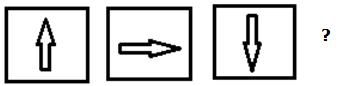Solution:
QUESTION: 8

Which number comes in between 39 and 41?

Solution:
QUESTION: 9

Which of the following shape is different from the other optins?

Solution:
QUESTION: 10

Complete the number series.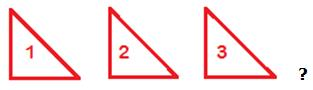Solution:
QUESTION: 11

A basket has space for keeping 10 eggs but only 4 eggs are kept in it. How many more eggs you can put in it?

Solution:

6 more eggs we can put in it.

QUESTION: 12

Identify the odd one out.

Solution:
QUESTION: 13

Complete the following serise.

Rs.100, Rs.200, Rs.300, Rs.400, Rs.500, Rs.?

Solution:
QUESTION: 14

Identify the missing number in the following series.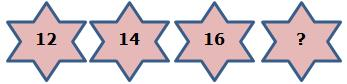Solution:
QUESTION: 15

Which of the following shape is different from the other opitions.

Solution:
QUESTION: 16

Complete the given series from the options given below.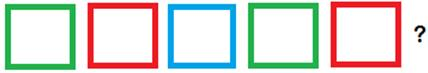Solution:
QUESTION: 17

Which is the smallest number in the follwoing options?

Solution:

A. 20, B. 25, C. 27,
A is correct.

QUESTION: 18

Which of the following series is not in order?

Solution:
QUESTION: 19

Complete the given series from the following options.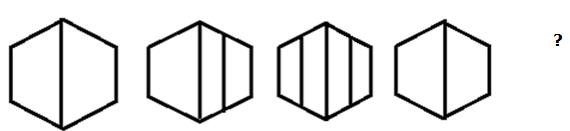Solution:
QUESTION: 20

Identify the missing number in the following ascending number order.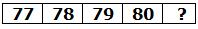Solution: